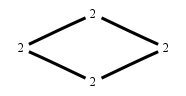# The Markov Bases Database

## C4_bin

The binary graphical model/graph model of C4.
The binary matroid model of rank 2 on 4 binary random variables with added columns 01, 10.

It is a hierarchical model of 4 variables. The dimension of the model is 8.The cardinality of the statespace is 16.

### Properties of the Markov basis

 Markov degree 4 16

 degree # of gen. 2 4 8 8

The model has the following properties:

• All variables are binary.
• It is a graph model and a graphical model.
• The minimal Markov basis is unique.
• The semigroup is normal.### FILES

 Markov basis: sufficient statistics matrix: C4_bin.mar (790 b) C4_bin.mat (534 b) C4_bin.mod (38 b) C4_bin.tar.gz (4.90 kb)

Wrong or missing information? Write us an email.

page last update on November 15 2020. ->Contact